# High School Math : How to find the volume of a sphere

## Example Questions

2 Next →

### Example Question #11 : How To Find The Volume Of A Sphere

The radius of a sphere is. What is the approximate volume of this sphere?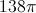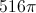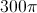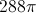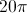Explanation: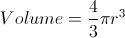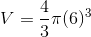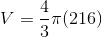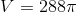### Example Question #21 : Spheres

A cube has a side dimension of 4. A sphere has a radius of 3. What is the volume of the two combined, if the cube is balanced on top of the sphere?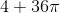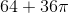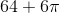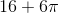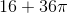Explanation: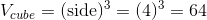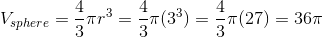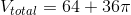### Example Question #1 : How To Find The Volume Of A Sphere

What is the volume of a sphere with a diameter of 6 in?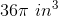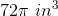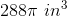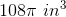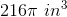Explanation:

The formula for the volume of a sphere is: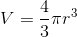where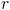= radius.  The diameter is 6 in, so the radius will be 3 in.

### Example Question #1941 : Hspt Mathematics

A solid hemisphere has a radius of length r. Let S be the number of square units, in terms of r, of the hemisphere's surface area. Let V be the number of cubic units, in terms of r, of the hemisphere's volume. What is the ratio of S to V?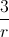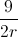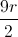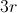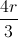Explanation:

First, let's find the surface area of the hemisphere. Because the hemisphere is basically a full sphere cut in half, we need to find half of the surface area of a full sphere. However, because the hemisphere also has a circular base, we must then add the area of the base.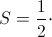(surface area of sphere) + (surface area of base)

The surface area of a sphere with radius r is equal to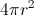. The surface area of the base is just equal to the surface area of a circle, which is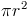.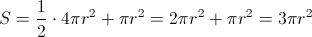The volume of the hemisphere is going to be half of the volume of an entire sphere. The volume for a full sphere is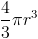.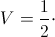(volume of sphere)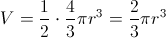Ultimately, the question asks us to find the ratio of S to V. To do this, we can write S to V as a fraction.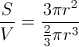In order to simplify this, let's multiply the numerator and denominator both by 3.=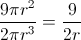The answer is.

### Example Question #11 : How To Find The Volume Of A Sphere

If the diameter of a sphere is, find the approximate volume of the sphere?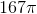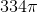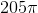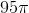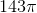Explanation:

The volume of a sphere =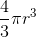Radius isof the diameter so the radius = 5.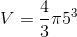or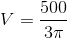which is approximately2 Next →

### All High School Math Resources